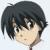mysql 外键不能关联到一自增的字段么？

【推荐阅读】滴滴出行的数据架构和信息流处理思路解析->>>error 1075 42000 incorrect table definition there can be only one auto column and it must be defined as a key

0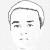0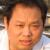0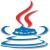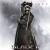00from django.db import models
from fields import BigIntegerAutoField

#python manage.py schemamigration author add_mobile_column --auto 执行model 修改 同步db的命令
#python manage.py migrate  提交 同步db
class Tucao(models.Model):
tcId = BigIntegerAutoField(primary_key=True,max_length=20,db_column='tucao_id',help_text='主键')
tcUserId = models.BigIntegerField(blank=False,max_length=11,db_column='tucao_account_id',help_text='吐嘈者帐户id,未登录者为-1')
tcAccountName = models.CharField(max_length=50,blank=False,db_column='tucao_account_name',help_text='吐嘈者昵称,匿名显示ip')
tcAccountEmail = models.EmailField(max_length=50,blank=False,db_column='tucao_account_email',help_text='吐嘈者email')
tcContext = models.TextField(max_length=160,blank=False,db_column='tucao_context',help_text='吐嘈内容')
tcPic = models.CharField(max_length=1000,blank=True,db_column='tucao_pic',help_text='吐槽图片链接')
class Meta:
db_table = 'tucao'
verbose_name = '吐槽表'

tcId = models.ForeignKey(Tucao,db_column='tucao_id',blank=True, null=True)
class Meta:
verbose_name = '吐槽回复表'0class BigIntegerAutoField(models.BigIntegerField):
def db_type(self, connection):
if 'mysql' in connection.__class__.__module__:
return 'bigint AUTO_INCREMENT'
return super(BigIntegerAutoField, self).db_type(connection)

class BigIntegerForeignKey(models.ForeignKey):
def __init__(self, to, to_field=None, **kwargs):
super(BigIntegerForeignKey, self).__init__(to, **kwargs)

def db_type(self, connection):
rel_field = self.rel.get_related_field()
if isinstance(rel_field, BigIntegerAutoField):
return models.BigIntegerField().db_type(connection=connection)
return rel_field.db_type(connection=connection)

tcId = BigIntegerForeignKey(Tucao,db_column='tucao_id',blank=True, null=True)3Q～～～ 还帮我写了代码。 不过我想弄清楚。 我之前那么写为什么不行呢。 我的BigIntegerAutoField 的代码就是按照你那么写的。
0